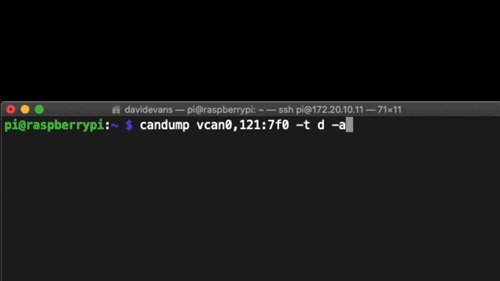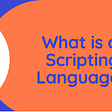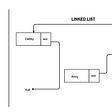# “Delete and Earn” problem using Dynamic Programming

In this article we will be discussing about one of the good problem related to Dynamic Programming which is one of the leetcode problem. Problem name is “Delete and Earn” which requires the concept of Arrays, Hash Table and Dynamic Programming. So lets start with the problem statement.

In the Problem statement we will be given an Integer Array ‘nums’ and we need to maximize the points that we get by performing two operations i.e. we can take any nums[i] and delete it to earn points. And after that we must delete every element that are equal to nums[i]-1 and every element equal to nums[i]+1.

After seeing that, we need to maximize the points then one thing that must come to our mind is Dynamic Programming. So the approach for this problem is :-

1. First we need to create an unordered_map ‘m’ to store the frequency of each number and we also find the maximum value present in nums array just for the sake of calculating the range of our for loop.
2. Then we need to create one array named ‘dp’ of max size that is 20001(taken from constraints) and store value in 0th index of dp array to the number of times zero appeared in nums array and the value of 1st index of dp array is set to the max value of dp, m*1. (Above steps notations are: dp=m and dp=max(dp,m*1))
3. Now the calculation of dynamic programming starts from here, first we run for loop from 2 till the maxi value that we have calculated earlier (we already set the 0th and 1st index of dp array that’s why we are starting from 2).
4. At each point the maximum score till now is dp[i-1] as we would not contribute to the score as it would had been deleted or it would be dp[i-2]+m[i]*i . We need to choose maximum of both in every step so that our prefix array i.e dp array should be filled completely.
5. At last we return the dp[maxi] which is our final answer.

Time Complexity of solution is:- O(N) or O(maxi)

Space Complexity of solution is:- O(N)

My Approach for the above problem is as follows (in C++ programming language):-

Hope the above solution will help to understand the problem more easily.

If anyone who is new to Dynamic Programming first he/she must think about the recursive approach and then think of what optimization that can be done in the solution to make it more efficient. This technique is known as Memoization technique which is a another way of implementing Dynamic Programming.

Hope this Article will be helpful to many for understanding the approach of this problem and for the beginners who were new to the dynamic programming concept.

--

--

--

## More from Yash Gaherwar

Computer Science Student at VIT, Pune

Love podcasts or audiobooks? Learn on the go with our new app.

## Coding Spring 2022## More Depth to Refactoring and Using Design Patterns## Launch AWS instance and attach volume using AWS CLI & script## CAN Bus Reverse Engineering With SocketCAN## What is a Scripting Language?## How I passed CKA in Oct’20## A Single Source of Truth / Eliminate Technical Debt## Yash Gaherwar

Computer Science Student at VIT, Pune

## More from Medium